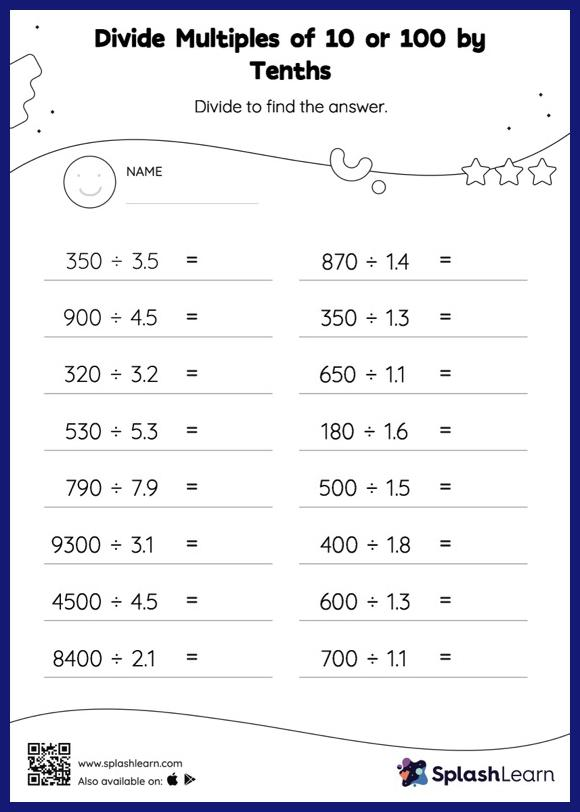# Divide Multiples of 10 or 100 by Tenths: Horizontal Division Worksheet

Home > Divide Multiples of 10 or 100 by Tenths: Horizontal DivisionChildren use their math skills to complete this divide multiples of 10 or 100 by tenths worksheet. Students divide a number by a decimal without taking the decimal point into account, and then they move each digit of the quotient to the left depending on how many decimal digits there are in the divisor. Divide multiples of 10 or 100 by tenths worksheet provides plenty of practice with this concept. In this worksheet, students practice solving problems written in the horizontal format. How numbers are laid out in a problem affects the method a student employs to solve it. Therefore to develop actual fluency and mastery of multiple strategies, students must practice different formats.